# TUTORIAL ON SUPERCUBE SOLVING (3) (Solving Even-Order Supercubes)

In this webpage, I will introduce how to solve even-order Supercubes. But before starting the introduction, I first need to point out two facts. First, since 2nd-order Supercubes do not have any center pieces and edge pieces, the solution method for these Supercubes is no different from that for 2nd-order Rubik's Cubes. Therefore in what follows I will only discuss the solution of Supercubes of the 4th-, 6th-, 8th-order, etc. Second, although even-order Supercubes have no core inner layer, they can be seen as odd-order Supercubes with the core inner layer hidden. For instance, a 4th-order Supercube can be seen as a 5th-order Supercube with the core inner layer hidden, as shown in the following diagram:By making use of this analogy between the odd-order and even-order Supercubes, we can apply the solution method of odd-order Supercubes to even-order Supercubes. But since even-order Supercubes have their own peculiarities, there will be some complications in the solution method. In what follows, I will focus on those aspects where the solution method of even-order Supercubes differs from that of odd-order Supercubes. Therefore, some parts of the following description of solution method will be quite brief, especially those aspects that are basically the same as solving odd-order Supercubes. If necessary, please refer to TUTORIAL ON SUPERCUBE SOLVING (2) (Solving Odd-Order Supercubes) for detailed description of each step.

## Step I: Match the Center Pieces on the first 4 Faces

This step is basically the same as Step I for solving odd-order Supercubes. The only difference in doing this step for even-order Supercubes is that since even-order Supercubes have no core center pieces, you can freely choose any face to be the blue face say. But after you make this first choice, the colors of the remaining faces are then determined.

## Step II: Match the Center Pieces on the 5th Face

This step is no different from Step II for solving odd-order Supercubes in that you need to use the following "(XYZ) Formulas" to match the center pieces on the 5th face:

 Clockwise (XYZ) Formula: Ux−1 R Uz−1 R−1 Ux R Uz R−1 (1) Anticlockwise (XYZ) Formula: Ux−1 R−1 Uz−1 R Ux R−1 Uz R (2)

## Step III: Match the Center Pieces on the 6th Face

Like Step III for solving odd-order Supercubes, this step is also sub-divided into three sub-steps.

### Sub-step 1: Check whether all Orbits of Non-core Center Pieces have Even Relative Parities

There are some peculiarities in doing this sub-step for even-order Supercubes. The first peculiarity concerns 4th-order Supercubes. Apart from point pieces, a 4th-order Supercube does not contain other center pieces. Remember that in TUTORIAL ON SUPERCUBE SOLVING (2) (Solving Odd-Order Supercubes), I have pointed out the following facts:

Fact 1: The relative parity of each point piece orbit is always even.

Fact 2: The orbits of paired pieces that are matched up with each other have the same relative parity.

Thanks to Fact 1, there is no need to do this step for 4th-order Supercubes.

Second, when doing this sub-step for an even-order Supercube with order higher than 4, there exists a problem. To understand this problem, please compare the 7th-order and the 6th-order Supercubes in the following diagram:When doing this sub-step for the 7th-order Supercube above, since the "A.00" core center piece has rotated by 90° clockwise, when you write out the alphabet list for each orbit of non-core center pieces, you should start from the bottom right hand corner of the red face (assuming that the red face is the 6th face). Thus, the alphabet lists of the "04" and "11" orbits are, respectively, "ADCB" and "CBAD", which have odd relative parities; whereas the alphabet list of the "10" orbit is "ABCD", which has even relative parity (note that you need not write out the alphabet lists of the "05" and "07" orbits because these two are orbits of point pieces, which must have even relative parities according to Fact 1. Neither do you need to write out the alphabet list of the "08" orbit because this orbit is matched up with the "04" orbit and so it must have the same relative parity as that of the "04" orbit, according to Fact 2).

When you try to do the same for the 6th-order Supercube above, you immediately encounter a problem. Since there is no core center piece in this Supercube, from which corner should you start when you write out the alphabet lists? In this case, the alphabet lists of the "05" and "07" orbits will give you useful hints. Note that Facts 1 and 2 given above are also applicable to even-order Supercubes. Now if you start from the top right hand corner of the red face, you will find that the alphabet lists of the "05" and "07" orbits are, respectively, "BCDA" and "DABC", which have odd relative parities, in violation of Fact 1. In fact, you can verify that if you start from the bottom left hand corner, you will also obtain odd relative parities. But if you start from the bottom right hand corner or top left hand corner, you will obtain even relative parities. Thus, the correct way to write out the alphabet lists is to start from the bottom right hand corner or top left hand corner of the red face. Now if you start from the bottom right hand corner, you will find that the alphabet lists of the "04" orbit is "ADCB", which has odd relative parity (by Fact 2, you may deduce that the "08" orbit also has odd relative parity).

In summary, when doing Sub-step 1 for an even-order Supercube, you should first identify a corner on the red face such that when you start writing the alphabet lists of the orbits of point pieces from that corner, you will obtain even relative parities. You then write out the alphabet lists of the paired pieces (note that even-order Supercubes have no cross pieces) and check whether all these alphabet lists have even relative parities. If so, then you may jump to Sub-step 3. If not, then you need to go through Sub-step 2.

### Sub-step 2: Ensure all Orbits of Non-core Center Pieces have Even Relative Parities

In TUTORIAL ON SUPERCUBE SOLVING (2) (Solving Odd-Order Supercubes), I have pointed out that when doing this sub-step for odd-order Supercubes, you only need to focus on the "focused pieces". These are the cross pieces in the right half of the red face. But since even-order Supercubes have no cross pieces, what are the focused pieces? Fortunately, there is a simple way to redefine the notion of "focused pieces" for even-order Supercubes. As mentioned above, even-order Supercubes can be seen as odd-order Supercubes with the core inner layer hidden. Therefore, the focused pieces for even-order Supercubes are just the center pieces that are immediately above the hidden cross pieces in the right half of the red face. For example, in the 6th-order Supercube shown above, the focused pieces are the "D.08" and "D.07" pieces encircled by the yellow frame in the following figure: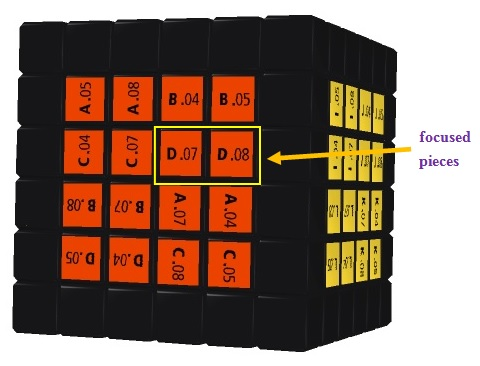To accomplish the objective of this sub-step, you first need to rotate the whole Supercube to make the red face facing upward, and then apply the following One Side Swapping Formula to every non-core inner layer on the right half of the red face that contains a focused piece orbit with odd relative parity:

One Side Swapping Formula: Lx−1 U2 Lx−1 U2 F2 Lx−1 F2 Rx U2 Rx−1 U2 Lx2     (3)

The subscripted variable x represents the non-core inner layer that you need to apply this formula to. Please note that by just focusing on the focused piece orbits as described above, you will autmoatically take care of all non-core center piece orbits, i.e. you will ensure that all non-core center piece orbits have even relative parities.

Using the 6th-order Supercube shown above as an example, you may recall that the "08" orbit has odd relative parity while the "07" orbit has even relative parity. This means that the first inner layer in the right half of the red face contains a focused piece orbit with odd relative parity. So you need to apply formula (3) to this inner layer, i.e. apply (3) once with the variable x substituted by 1. (Note that the leftmost center piece among the focused pieces, e.g. the "D.07" piece in the above example, is necessarily a point piece. By Fact 1 above, its orbit must have even relative parity and so need not be considered. For this reason, you can always exclude the leftmost center piece among the focused pieces from consideration.) After the above operation, all orbits of non-core center pieces in this Supercube will have even relative parities.

### Sub-step 3: Use the (XYZ) Formulas to restore the Non-core Center Piece Orbits to Normal

This sub-step is no different from Sub-step 3 for solving odd-order Supercubes in that you need to use the (XYZ) Formulas (i.e. formulas (1) and (2) given above) and Table 1 below to restore the alphabet list of each non-core center piece orbit to "ABCD".

Table 1
Alphabet ListRestoration Procedure
ABCD
no need to restore
ABDC
odd relative parity
ACBD
odd relative parity
ACDB
(B#D) → (#BC)
(D#B) → (#DC)
odd relative parity
BACD
odd relative parity
(A#B) → (DAC) → (#DA)
(A#C) → (#AB)
BCDA
odd relative parity
BDAC
odd relative parity
BDCA
(D#B) → (#DA)
CABD
(C#A) → (#CB)
odd relative parity
odd relative parity
CBDA
(C#A) → (#CD)
CDAB
(A#B) → (#AC) → (D#B) → (#DC)
CDBA
odd relative parity
DABC
odd relative parity
DACB
(B#D) → (#BA)
DBAC
(C#A) → (#CD)
DBCA
odd relative parity
DCAB
odd relative parity
DCBA
(A#D) → (CA#) → (#CB)

## Step IV: Match the Edge Pieces on the first 10 Edges

This step is no different from Step IV for solving odd-order Supercubes. No additional explanation is needed.

## Step V: Match the Edge Pieces on the remaining 2 Edges

This step is similar to Step V for solving odd-order Supercubes in that you may need to use some formulas to swap / flip some edge pieces. These include the One Side Swapping Formula given above, i.e. formula (3) and the following three formulas:

Two Side Swapping Formula: One Side Flipping Formula: F2 Lx−1 F B−1 R2 F−1 B Rx Lx F B−1 R2 F−1 B Rx−1 F2 (4) Rx2 B2 U2 Lx U2 Rx−1 U2 Rx U2 F2 Rx F2 Lx−1 B2 Rx2 (5) U−1 F R−1 U F−1 Rx−1 Lx F U−1 R F−1 U Rx Lx−1 (6)

Note that you do not need to use the "Core Edge Flipping Formula" in this step because even-order Supercubes do not have core edge pieces. For this reason, this formula is not given here.

Similar to the case of solving odd-order Supercubes, you need not worry that by using formulas (3) - (6), you may screw up the centers that have been matched in the previous steps, because formulas (4) and (6) will not screw up any center (try and see), while formulas (3) and (5) are always used under two possible situations when solving even-order Supercubes. The first possible situation is that formulas (3) and (5) are used one after the other on the same non-core inner layer. This situation is illustrated by the following figure: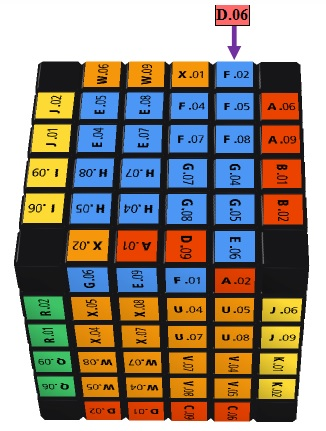To match the edge pieces on the front-top and back-top edges of the Supercube shown above, you may first substitute 1 into the variable x and use formula (5). This will flip and swap the "G.06-X.02" and "A.02-E.06" edge pieces. You then substitute 1 into the variable x and use formula (3). This will swap the "D.06-F.02" and "G.06-X.02" edge pieces and thus yield the desired result. Note that by using formulas (3) and (5) one after the other on the same non-core inner layer, the net effect is that the top center (as well as other centers) will not get screwed up (try and see).

The second possible situation is that either formula (3) or (5) is used on each non-core inner layer in the right (or left) half of the Supercube. This situation is illustrated by the following figure: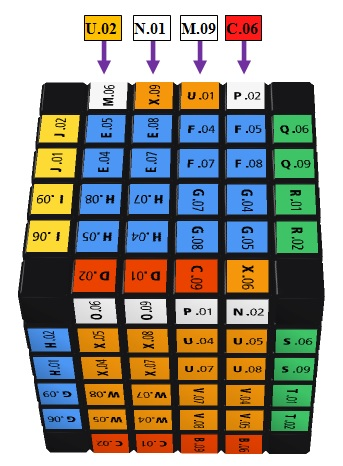To match the edge pieces on the front-top and back-top edges of the Supercube shown above, you may first substitute 1 into the variable x and use formula (3). This will swap the "C.06-P.02" and "N.02-X.06" edge pieces. Next you rotate the whole Supercube so that the orange face now faces backward. You then substitute 2 into the variable x and use formula (5). This will flip and swap the "N.01-X.09" and "M.09-U.01" edge pieces and thus yield the desired result. Note that by using either formula (3) or (5) on each non-core inner layer in the right (or left) half of the Supercube, the net effect is that the top center (as well as other centers) will not get screwed up (try and see).

## Step VI: Solve the Corners and Edges

Just like the case of odd-order Supercubes, in this step you use the same method of solving a 3rd order Rubik's Cube to solve the corners and edges of even-order Supercubes. But since even-order Supercubes have their own peculiarities, there are some special features that are different from the method of solving a 3rd order Rubik's Cube. To faciliate discussion below, I will list the seven sub-steps of this step below. These sub-steps corresponds to the seven steps introduced in 7 Steps Solution Guide:

### Sub-step 1: Solve the Bottom Edges Sub-step 2: Solve the Bottom Corners Sub-step 3: Solve the Middle Layer Edges Sub-step 4: Orient the Top Edges correctly Sub-step 5: Place the Top Edges correctly Sub-step 6: Place the Top Corners correctly Sub-step 7: Orient the Top Corners correctly

Here "place correctly" means placing a piece at the correct position, "orient correctly" means making the facelets of a piece face the correct directions so that there is no twisting (of a corner piece) and no flipping (of an edge piece), whereas "solve" means both "place correctly" and "orient correctly".

For most of the sub-steps listed above, there is no difference between the methods of solving odd-order and even-order Supercubes. The only exceptions are Sub-steps 4 and 6, which I will discuss below.

### Sub-step 4: Orient the Top Edges correctly

For odd-order Supercubes, before doing this sub-step, there can be 0 or 2 top edges oriented correctly if not all of them are oriented correctly, and you can accomplish the objective of this sub-step by using the formula FRUR−1U−1F−1 given in 7 Steps Solution Guide. However, for even-order Supercubes, the situation is more complicated. Before doing this sub-step, there can be 0, 1, 2 or 3 top edges oriented correctly if not all of them are oriented correctly. I use the following figure to illustrate this point: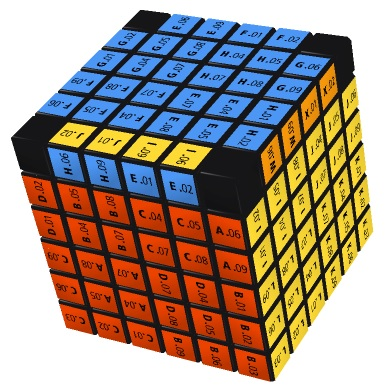The above figure shows a 6th-order Supercube. Among the 4 edges on the top face of this Supercube, only the "yellow-blue" edge is oriented incorrectly with its blue facelet facing forward instead of upward (note that although the "orange-blue" edge is placed at the incorrect position, it is oriented correctly with its blue facelet facing upward). In this case, if you keep on using the formula FRUR−1U−1F−1, you can never finish this sub-step. What you need to do is rotate the whole Supercube so that the incorrect edge is facing forward and then apply the One Side Flipping Formula (5) to flip the incorrect edge (since there are two inner layers in the right half of this 6th-order Supercube, you need to apply (5) to both of these inner layers). After applying (5), you finish this sub-step.

In case there is only 1 top edge oriented correctly before doing this sub-step, you can also apply (5). The result of this move is a Supercube with 0 or 2 top edges oriented correctly. You can then use the formula FRUR−1U−1F−1 to finish this sub-step.

### Sub-step 6: Place the Top Corners correctly

For odd-order Supercubes, before doing this sub-step, there can be 0 or 1 top corner placed correctly if not all of them are placed correctly, and you can accomplish the objective of this sub-step by using the formula URU−1L−1UR−1U−1L given in 7 Steps Solution Guide. However, for even-order Supercubes, the situation is more complicated. Before doing this sub-step, there can be 0, 1 or 2 top corners placed correctly if not all of them are placed correctly. Moreover, in case exactly 2 top corners are placed correctly, the two correct corners may lie on a vertical / horizontal line or they may lie on a diagonal. I use the following figure to illustrate this point: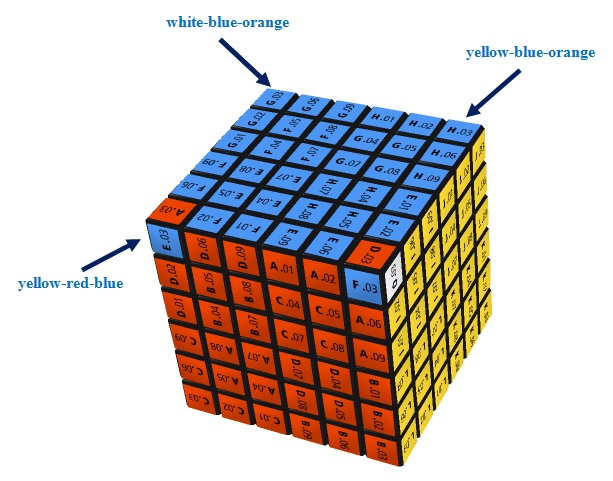The above figure shows a 6th-order Supercube. Among the 4 corner pieces on the top face of this Supercube, exactly two (i.e. the "yellow-blue-orange" and "white-blue-orange" corner pieces) are placed correctly and they lie on a horizontal line. In this case, if you keep on using the formula URU−1L−1UR−1U−1L, you can never finish this sub-step. What you need to do is rotate the whole Supercube so that the two correct corner pieces are at the "front-right-top" and "back-right-top" corners. You then apply the Two Side Swapping Formula (4) and Two Side Flipping Formula (6) successively. By so doing, you will screw up the 4 top edges that have been placed correctly in Sub-step 5. So you need to do Sub-step 5 again. After this, you will find that there is only 1 top corner placed correctly. You can then use the formula URU−1L−1UR−1U−1L to finish this sub-step.

In case exactly 2 top corners are placed correctly and they lie on a diagonal, you can rotate the whole Supercube so that one of the correct corner pieces is at the "front-right-top" corner. After applying URU−1L−1UR−1U−1L once, the result is a Supercube with exactly 2 top corners placed correctly and lying on a vertical / horizontal line. You may then proceed as described above.

In case no top corners are placed correctly, just apply URU−1L−1UR−1U−1L once or twice. The result is a Supercube with exactly 1 or 2 top corners placed correctly. You may then proceed as described above.

## Step VII: Orient the Centers correctly

This step is no different from Step VII for solving odd-order Supercubes in that you need to use the following formulas to orient the centers correctly:

 Two Center Rotation Formula: L−1 R F−1 B U−1 D Ln D−1 U B−1 F R−1 L U−n (7) One Center Rotation Formula: (U R L U2 R−1 L−1)2 (8)

Go to Supercube Links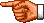Go toMy Personal Homepage (English Version)
Go toMy Personal Homepage (Chinese Version)